Next: Simple Pendula Up: Simple Harmonic Oscillation Previous: Simple Harmonic Oscillator Equation

# LC Circuits

Consider an electrical circuit consisting of an inductor, of inductance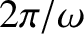, connected in series with a capacitor, of capacitance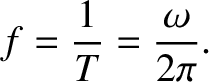. (See Figure 4.) Such a circuit is known as an LC circuit, for obvious reasons. Suppose that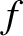is the instantaneous current flowing around the circuit. According to standard electrical circuit theory (Fitzpatrick 2008), the potential drop across the inductor (in the direction of the current flow) is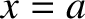. Again, from standard electrical circuit theory (ibid.), the potential drop across the capacitor is, whereis the charge stored on the capacitor's positive plate. However, because electric charge is a conserved quantity (ibid.), the current flowing around the circuit is equal to the rate at which charge accumulates on the capacitor's positive plate: that is,. According to Kirchhoff's second circuital law, the sum of the potential drops across the various components of a closed circuital loop is equal to zero (Grant and Philips 1975). In other words,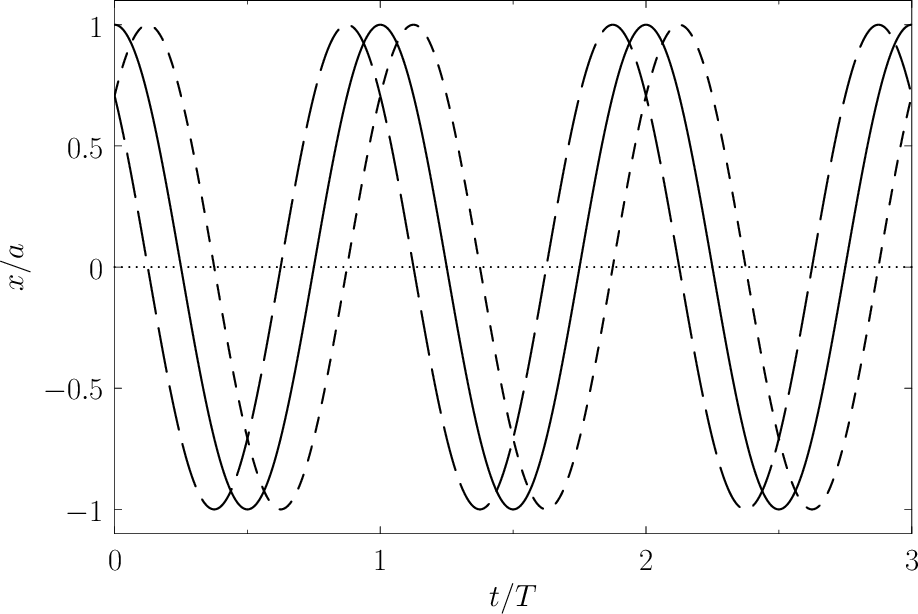(33)

Dividing by, and differentiating with respect to, we obtain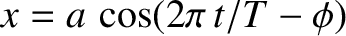(34)

where(35)

Comparison with Equation (17) reveals that Equation (34) is a simple harmonic oscillator equation with the associated angular oscillation frequency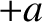. We conclude that the current in an LC circuit executes simple harmonic oscillations of the form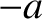(36)

where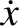and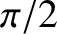are constants. According to Equation (33), the potential drop,, across the capacitor is minus that across the inductor, so that, giving(37)

Here, use has been made of the trigonometric identity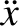. (See Appendix B.) It follows that the voltage in an LC circuit oscillates at the same frequency as the current, but with a phase shift of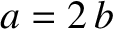radians. In other words, the voltage is maximal when the current is zero, and vice versa. The amplitude of the voltage oscillation is that of the current oscillation multiplied by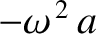. Thus, we can also write(38)Comparing with Equation (22), we deduce that(39)

is a conserved quantity. However,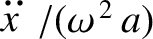, and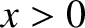. Thus, multiplying the preceding expression by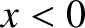, we obtain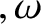(40)

The first and second terms on the right-hand side of the preceding expression can be recognized as the instantaneous energies stored in the capacitor and the inductor, respectively (Fitzpatrick 2008). The former energy is stored in the electric field generated when the capacitor is charged, whereas the latter is stored in the magnetic field induced when current flows through the inductor. It follows that that the quantityin Equation (40) is the total energy of the circuit, and that this energy is a conserved quantity. The oscillations of an LC circuit can, thus, be understood as a cyclic interchange between electric energy stored in the capacitor, and magnetic energy stored in the inductor.

Suppose that at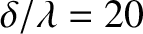the capacitor is charged to a voltage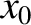, and there is zero current flowing through the inductor. In other words, the initial state is one in which all of the circuit energy resides in the capacitor. The initial conditions are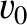and. In this case, it can be shown that the current evolves in time as(41)

Suppose that atthe capacitor is fully discharged, and there is a currentflowing through the inductor. In other words, the initial state is one in which all of the circuit energy resides in the inductor. The initial conditions areand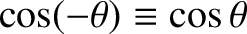. In this case, it can be demonstrated that the current evolves in time as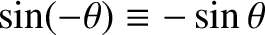(42)

Suppose, finally, that atthe capacitor is charged to a voltage, and the current flowing through the inductor is. Since the solutions of the simple harmonic oscillator equation are superposable, it follows that the current evolves in time as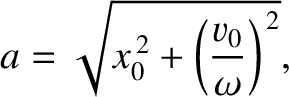(43)

Furthermore, according to Equation (38), the voltage evolves in time as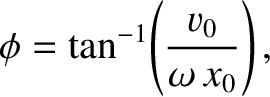(44)

or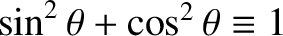(45)

Here, use has been made of the trigonometric identitiesand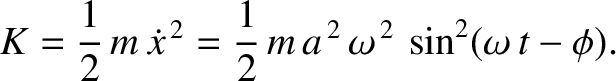. (See Appendix B.)

The instantaneous electrical power absorption by the capacitor, which can be shown to be minus the instantaneous power absorption by the inductor, is (Fitzpatrick 2008)(46)

where use has been made of Equations (43) and (45), as well as the trigonometric identities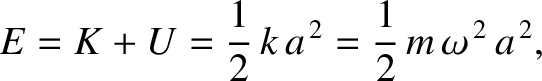and. (See Appendix B.) Hence, the average power absorption during a cycle of the oscillation,(47)

is zero, because it is readily demonstrated that. In other words, any energy that the capacitor absorbs from the circuit during one half of the oscillation cycle is returned to the circuit, without loss, during the another. The same goes for the inductor.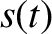Next: Simple Pendula Up: Simple Harmonic Oscillation Previous: Simple Harmonic Oscillator Equation
Richard Fitzpatrick 2013-04-08